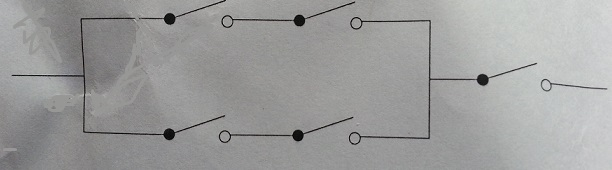# Heisenberg Electric Circuit

Probability Level 3In the above circuit, the switches operate independently of each other. Each switch is closed with probability $\frac{1}{3}$. The circuit will work, if there is a closed path from the left to the right.

What is the probability that the circuit is working?

×

Problem Loading...

Note Loading...

Set Loading...